Reach Us+44-1474-556909
On the Relevance of Stochastic Controls | OMICS International
Journal of Applied & Computational Mathematics
All submissions of the EM system will be redirected to Online Manuscript Submission System. Authors are requested to submit articles directly to Online Manuscript Submission System of respective journal.

# On the Relevance of Stochastic Controls

Orlando Gomes*

Lisbon School of Accounting and Administration (ISCAL-IPL) and Business Research Unit of the Lisbon University Institute (BRU/LUI), Portugal

*Corresponding Author:
Orlando Gomes
Lisbon School of Accounting and Administration (ISCAL-IPL) and Business Research
Unit of the Lisbon University Institute (BRU/LUI), Portugal
Tel: 351-933420915
E-mail: [email protected]

Received August 18, 2014; Accepted August 20, 2014; Published August 26, 2014

Citation: Gomes O (2014) On the Relevance of Stochastic Controls. J Appl Computat Math 3:181. doi: 10.4172/2168-9679.1000181

Copyright: 2014 Gomes O. This is an open-access article distributed under the terms of the Creative Commons Attribution License, which permits unrestricted use, distribution, and reproduction in any medium, provided the original author and source are credited.

Visit for more related articles at Journal of Applied & Computational Mathematics

#### Abstract

Many scientifically relevant problems share two salient features. First, they are dynamic; second, they involve uncertainty. Hence, it is natural that researchers would be concerned with the study of events taking place in stochastic dynamic settings.

#### Overview

Many scientifically relevant problems share two salient features. First, they are dynamic; second, they involve uncertainty. Hence, it is natural that researchers would be concerned with the study of events taking place in stochastic dynamic settings. In these settings, the decision-maker will typically want to select optimal paths for an array of control variables in order to maximize or minimize the current value of a sequence of future expected outcomes. In this article, we defend the argument that exploring techniques and applications in the field of stochastic optimal control theory is vital for the advancement of applied science. Although solid steps have been taken, in the last few years, to consolidate the theory of stochastic controls and to make this an adequate tool to address many important problems in multiple fields of knowledge, further work is still necessary to accomplish new insights and to unveil new results in an area of extreme complexity, where the search for efficient paths is many times hampered by the high degree of underlying uncertainty.

The benchmark optimization problem

Stochastic optimal control problems are concerned with the intertemporal optimization (maximization or minimization) of an objective function subject to one or more constraints that, in continuous time, acquire the form of stochastic differential equations. The objective function typically respects to the expected value of a sequence of utility levels that range from the initial date t=0 to some future horizon. In the case of economic problems, the horizon is commonly assumed as infinite and the future is discounted at a constant rate ρ>0. Taking the autonomous case, for which time is not an explicit argument of the problem’s functional, the objective function acquires the following shape,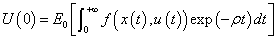(1)

Function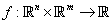is assumed to be a real-valued continuous and differentiable function. Two categories of variables constitute the arguments of f, namely the state variables,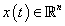and the control variables,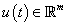. State variables are those that have their laws of motion determined by the differential equations respecting to the problem’s constraints; control variables are the ones that the decision-maker is able to control in order to pursue the specified dynamic goal.

The constraints underlying the optimization problem are, as mentioned above, stochastic differential equations. A generic specification is the following,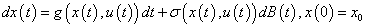(5)

In equation (2),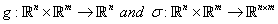. The In control problems involving a constant discount of future rewards and an infinite horizon are designated as reinforcement learning problems.term B(t) is an m-dimensional stochastic process defined in a filtered probability space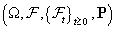Frequently, the stochastic process takes the form of a Wiener process or Brownian motion. The formal definition of Brownian motion is as follows

If, for all 0≤s<t, B(t)-B(s) is independent of the stopping time σ-algebra Fs and is normally distributed with mean 0 and variancecovariance matrix (t-s)I, with I an m×m identity matrix, then B(t) is a Brownian motion. The two mentioned properties are presentable in the form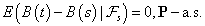(8)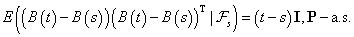(9)

Roughly speaking, one might say that a Brownian motion is a normally distributed stochastic process with stationary independent increments. By adding the Brownian motion to an otherwise multidimensional deterministic differential equation, the time trajectories of the problem’s endogenous variables will no longer correspond to deterministic paths; instead, the trajectories will exhibit persistent fluctuations around the non-stochastic trend. Instead of a Brownian motion, one may consider other types of stochastic processes in order to build a stochastic differential equation. A popular alternative is a Poisson process. A Poisson process, also known as counting process or pure jump process, is a stochastic process q(t) such that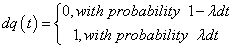(10)

Parameter λ>0 is designated arrival rate. The Poisson process implies that the state variables in the stochastic differential equations are subject to jumps at random dates. This kind of process is useful to model phenomena as technological progress, where uncertainty concerning the arrival date of new innovations and about the extent of such innovations exists. Other types of, more sophisticated, stochastic processes might also be included in the constraints of the optimal control problem, namely Lévy processes, which combine features of the Brownian motion and of the Poisson process2.

Independently of the stochastic process that best describes the type of randomness associated to the evolution of the assumed state variables, the optimal control stochastic problem will typically correspond to the maximization of U(0) as given by expression (1) subject to a series of n stochastic constraints, as those in equation (2). In its generic form, this is not a simple problem to solve. Nevertheless, under some simplifications and constraints, this framework is able to deliver meaningful results that answer challenging questions that are posed by events taking place in nature, in the society and in the economy.

#### A Brief Note on General Solution Techniques

The most common technique to approach and solve stochastic optimal control problems consists in the construction of the respective Hamilton-Jacobi-Bellman (HJB) equation, which applies to the optimization problem in its generic form.

Let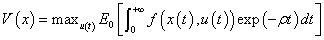(11)

Given V(x), the HJB equation takes the form of a non-linear ordinary differential equation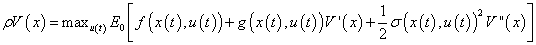(12)

HJB equations are not always tractable from an analytical point of view, namely when the dimensionality of the underlying system is high or when the problem involves nonlinear constraints. The general stochastic control problem, as presented above, is, in fact, intractable to solve, requiring an unreasonable quantity of computational resources. Common algorithms demand millions of iterations for a task to be learned in the context of such generic problem.

Recent relevant advancements in the treatment of stochastic controls have allowed, on one hand, for a deeper understanding of the implications of the general problem and, on the other hand, for a rigorous and detailed analysis of some meaningful particular cases. For instance, Horowitz  takes a more insightful look at the HJB equation and develops algorithms that are capable of dealing directly with the optimal solutions of high dimensional nonlinear systems. Previously, Kappen et al., Todorov et al., and Theodorou et al., [2-5] have discovered that particular assumptions on the structure of the dynamic system make it possible to transform the HJB equation into a linear equation, allowing for analytical tractability. Specifically, the work by Kappen [2,3] indicates that an efficient solution is attainable when the control problem is defined in a finite horizon, the control term is linear and additive and the cost of the control is quadratic. Another form of approaching stochastic optimal control problems in order to deliver important insights is through state space discretization, what transforms the problem into a Markov decision process. In discrete time, the problem becomes easier to deal with, since it avoids the consideration of partial differential equations, which are typically difficult to analyze and often imply the impossibility of determining explicit analytical results .

#### Some Specific Applications and Contributions

Stochastic control techniques may be applied to any inter-temporal optimization problem for which the decision-maker has the faculty of controlling partially the environment and chooses to attain a specific goal. In Kappen , control problems are associated with animal behavior. Living organisms, including human beings, employ cognitive resources to take decisions. Frequently, these are not static decisions what implies the need to revisit the problem at successive time periods. Therefore, there is a recurrent process of adaptation and learning. Control theory studies how living organisms optimize a sequence of actions to attain inter-temporal goals. Because future events are not known with certainty, the optimization process implies the assumption of a probabilistic model of the expected outcomes. At each time step, given the uncertain outcome, the agent must re-estimate the trajectories of the control variables, and in this way one might state that there is a close link between adaptation and learning, on one hand, and stochastic optimization, on the other. Besides individual decision-making that takes place at the level of the brain, stochastic control might also be applied to scenarios where multiple agents have to solve a task. Besides optimization in time, this setting requires also the optimal coordination of the agents’ actions. An example of this type of problem and the exploration of the respective outcomes is offered by Wiegerinck et al. . The problem is one in which there is a common goal but where agents act in a decentralized way, choosing the paths that desirably lead to an optimal distribution of the agents across a given number of targets

The prototypical example concerning multi-agent optimal control presented in the mentioned paper respects to a problem of firemen allocation across a number of active fires. Stochastic control techniques allow encountering a solution that is optimal from the social point of view, i.e., a solution such that firemen do not tend to concentrate in the same fires; on the contrary, they are able to distribute themselves in the direction of different final locations just by observing the trajectories followed by others. This is an interesting problem because it can be straightforwardly adapted to many situations that arise in society and in the economy: although agents behave on their own interest, their ultimate goal can only be accomplished by maximizing the performance of the group or the team. For instance, one could extend this framework in order to explain how market relations are organized or how different individuals choose different transportation to commute in an urban area. In fact, optimal control allows dealing with every stochastic environment in which agents have to distribute themselves efficiently over a number of targets. Again, the complexity of the problem emerges from the underlying uncertainty: in a stochastic environment, a configuration that is apparently optimal at t=0 may no longer be optimal at a future date.

In the field of economics, one of the most popular applications of stochastic optimal control is the one proposed by Merton . In Merton’s model, a representative consumer selects efficient consumption and portfolio investment strategies over a long time horizon. Uncertainty is, in this case, associated to a risky asset for which the expected return and the volatility are estimated. This model basically consists in the adaptation of the Ramsey model, i.e., of the utility maximization problem of the representative agent facing a resource constraint, to a stochastic environment.

A related area where stochastic controls have been applied with success is neoclassical growth theory. In a stochastic growth model, the standard benchmark framework is modified in order to account for uncertainty in technological progress. As in the deterministic case, growth can be explained through the analysis of a capital accumulation differential equation, but now this becomes a stochastic differential equation Brock et al., and Merton et al., for the analytical treatment of this model [10,11].

#### Why Stochastic Controls?

As highlighted by Horowitz , in control theory the ultimate objective is to direct the system under appreciation towards a specified goal. The system involves laws of motion for the state variables, which constrain the choices of the agent solving the problem. The agent has, however, at her disposal, a set of control variables that constitute signals that she may manipulate in order to achieve the intended goals. Control theory is, then, concerned with designing efficient and robust solutions for the problem at hand, i.e., it is concerned with defining optimal time trajectories for the available control variables. At a first glance, optimal control problems do not seem too difficult to approach. In theory, one knows the initial state of the system, the inter-temporal goal to fulfill and the constraints that are faced by the problem solver. The fundamental point, though, is that one is dealing with the future; the plan is set now, at t=0, for an horizon that starts at t=0 and extends to a pre-defined future date. Therefore, as emphasized by Kappen , the control problem is stochastic in nature. There is uncertainty associated with future outcomes and the best the agent can do is to compute the optimal trajectory of some control variable(s) contingent on how the system is supposed to evolve. It is the stochastic element that sophisticates the problem and makes it hard to approach.

A deterministic plan can be solved at the initial period and never revised, because nothing in the environment will presumably change. Stochastic controls require a permanent revaluation of the dynamic conditions as these potentially depart from expected values. When evolving from determinism to stochasticity one loses in tractability but one surely gains in realism. The relevance of approaching plans through the lenses of stochastic optimal control is not exhausted in the determination of optimal paths. It is also important to guarantee their stability. Stabilization methods in the presence of uncertainty must also be an issue in mind when addressing this type of problems. This is also a concern that optimal control theorists have, as highlighted by Horowitz .

In synthesis, stochastic dynamic optimization problems are a significant part of the decision problems one finds in nature, in the society and in the economy. As a consequence, it is undoubtedly of extreme relevance to continue to build the tools and to explore the applications that somehow fall in the extremely rich scientific domain that is stochastic controls

#### Literature

The modern theory of optimal control starts with Bellman et al. ; it is also Bellman  who presents the first results on stochastic controls. Since then, many important results have come to light. Today, there is a vast literature on stochastic optimal control, which is synthesized in some volumes that constitute the main references in this area. These include, just to cite a few, Kamien et al. , Yong and Zhou , Kendrick , Bertsekas [17,18] and Oksendal and Sulem . Walde [20-22] offers a comprehensive presentation of dynamic optimization models in economics. In four parts, the book addresses deterministic optimization in discrete and continuous time and stochastic optimization in discrete and continuous time. Concerning stochastic optimal control problems in continuous time, it is explained, in this manuscript, in a simple mode, what a stochastic process is, how a stochastic process might integrate a stochastic differential equation and how the shape of the stochastic differential equation depends on the type of stochastic process (Brownian motion, Poisson process or Lévy process). A similar discussion may be found in Brito .

#### References

Select your language of interest to view the total content in your interested language

### Article Usage

• Total views: 11996
• [From(publication date):
October-2014 - Sep 23, 2019]
• Breakdown by view type
• HTML page views : 8196

## Post your commentCan't read the image? click here to refresh
###### Peer Reviewed Journals

Make the best use of Scientific Research and information from our 700 + peer reviewed, Open Access Journals

International Conferences 2019-20

Meet Inspiring Speakers and Experts at our 3000+ Global Annual Meetings

Top